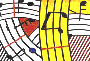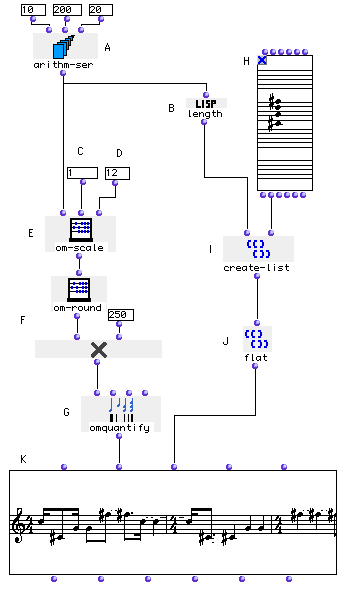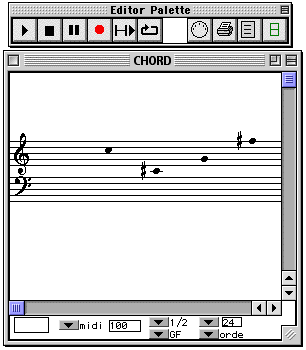Tutorial 30 - Operation on lists I

Generation of lists with identical length

Topics

arithm-ser, CHORD, create-list, flat, length, om-round, om-scale, om-quantify, om* and VOICE.Description
This example creates a list of notes (L) containing the same number of elements as a given list of durations (I) which are derived from an arithmetical series (A).

Patch structure

A: The arithm-ser box (A) creates an arithmetical series from value 10 to value 200 with a step of 20.

B: The length box (B), as we have already seen, counts the number of elements in the list coming out of arithm-ser box (A).

C: The value you enter in this input module (C) will correspond to the shortest duration in your rhythmic sequence( It is of course an arbitrary number which will be scaled afterwards).

D: The value you enter in this input module (D) will correspond to the longest duration in your rhythmic sequence.

E: The module om-scale (E) scales the values coming out of the arithm-ser box (A) between the values entered in inputs (C) and (D). In order to round the result we will use om-round. There we will be sure to have integers going from 1 to 12.

F: The om* module will multiply each element of the list coming out from om-round by 250 in order to output a correct format ofdurations in milliseconds (250 representing a sixteenth note).

G: The om-quantify (G) quantifies the list of values coming out of om-round into measures of 4/4, with a tempo of 60, and with a maximum unit division of 8 (thirty-second-note resolution).These a re the default values.H: Open and edit a sequence of notes in the CHORD box (H) (using the "order" mode). Then close the box and lock it.

I: The create-list box (I) makes a list with the elements you have entered in the CHORD box (H). The length of the list is defined by the value coming out of the length box (B).

J: The flat box (J) transforms the incoming list of lists into a flat list (a simple list).

K: Evaluate the VOICE box (K) and open it to see the result. Change either the values of the arithm-ser box, the values of the inputs numbox (C) and (D) and look at the new results.